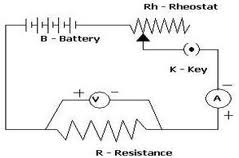# How to verify Ohm’s Law

We already know the statement of Ohm’s Law which is

“If the physical state of the conductor (Temperature and mechanical strain etc.) remains unchanged, then current flowing through a conductor is always directly proportional to the potential difference across the two ends of the conductor”

Mathematically

V=IR

where constant of proportionality R is called the electric resistance or simply resistance of the conductor.

Ohm’s Law can be verified is we manage to show that for a given conductor (piece of wire) the ratio V:I is constant. Resistance of the conductor is a constant for a known temperature range.

To verify this law you can set up a circuit with resistors of fixed values, a variable voltage source, a voltmeter and an ammeter as shown below in the figureTake one resistor, connect a voltmeter parallel to it and an ammeter in series to the resistor and the voltage source. Change the voltage; take the reading from the voltmeter and the corresponding current reading from the ammeter. Plot a graph with voltages along X axis and current along Y axis. You should get a straight line of the form y = mx

Repeat the same taking different values of resistors. ‘m’ will be different for different resistances. The Ohm’s will be verified if for each values of resistances, the V-I graph is of the form y = mx. That is a straight line passing through the origin, making an angle with the axes.

### 5 Leave a Reply

5 Comment threads
0 Thread replies
0 Followers

Most reacted comment
Hottest comment thread
0 Comment authors

This site uses Akismet to reduce spam. Learn how your comment data is processed.

Subscribe
Notify of

ram

Thnx

i will try to do the practical, I hope the circuit will work

thanks

thanks